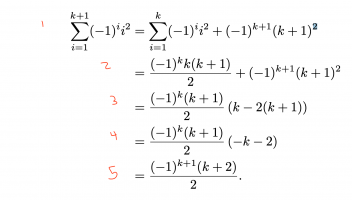# Gaps in Inductive Proof

#### gappy

##### New member
Hi,

Context
I am not traditionally a math student, but I am studying some math-intensive subjects. It's a bit odd, but I understand parts of much of the math we do while also having gaps in what I feel is probably pretty basic stuff. Many of these gaps seem to surface in the proofs we have to do. I am trying to practice proofs, but, beyond simple proofs, there are often leaps that I am unable to reconcile. The below is not homework, but extra practice I am trying to understand.

Question
Below is part of an inductive proof. I understand the equivalency stated at line 1 as well as the transition from line 1 to line 2. However, how do we get from line 2 to line 3? Additionally, I understand the transition from line 3 to line 4 but what steps are taken to move from line 4 to line 5?Does anyone also have any suggestions for resources that may help me understand the steps that can be taken when writing proofs? I generally know where I am starting and where I am going. I also have a vague, sometimes more than a vague, idea of how to get from the start to finish, but often get lost when it comes to details or procedure. Most likely from lack of knowledge of relationships such as those in the steps above.

I appreciate any help or guidance.

#### JeffM

##### Elite Member
Line 2 to 3 leaves out a lot of steps, but it is basic algebra.

$\dfrac{(-1)^k k(k + 1)}{2} + (-1)^{(k + 1)}(k + 1)^2 = \dfrac{1}{2} * \left \{ (-1)^k k(k + 1) + 2(-1)^{(k+1)}(k + 1)^2 \right \} =$
$\dfrac{(k + 1)}{2} * \{(-1)^k * k + 2(-1)^{(k+1)}(k + 1) \} = \dfrac{(k + 1)}{2} * \{(-1)^k k + 2(-1)^k(-1)^1(k + 1)\} =$
$\dfrac{(-1)^k(k + 1)}{2} * \{k + 2(-1)(k + 1)\} = \dfrac{(-1)^k(k + 1)}{2} * \{k - 2(k + 1)\}.$

#### lev888

##### Senior Member
From 4 to 5:
(-k-2) is (-1)(k+2).
(-1) is multiplied by (-1)^k, which becomes (-1)^(k+1).
(k+2) is in the numerator, as expected. But what happens to (k+1) that was in the numerator, I don't know.

•JeffM

#### JeffM

##### Elite Member
From 4 to 5:
(-k-2) is (-1)(k+2).
(-1) is multiplied by (-1)^k, which becomes (-1)^(k+1).
(k+2) is in the numerator, as expected. But what happens to (k+1) that was in the numerator, I don't know.
I think that is an error.

It looks to me as though the inductive hypothesis (a term I hate) is

$\sum_{i=1}^k (-1)^i i^2 = \dfrac{(-1)^kk(k + 1)}{2}.$.

A correct transition from 4 to 5 gives (as you implied):

$\dfrac{(-1)^{(k+1)}(k + 1)(k + 2)}{2} = \dfrac{(-1)^{(k+1)}(k + 1)\{(k + 1) + 1\}}{2}.$

#### JeffM

##### Elite Member
@gappy

In inductive proofs, it helps to write down at the beginning where you want to end up (the final k + 1 result). Each step should move toward that goal. It will help you understand examples and find your own proofs.

With that said, I think the given proof leaves out too many steps for a beginning student, would be clearer with more grouping and operator symbols, and then makes a mistake. Hard to learn from that kind of example.

I suggest in the future that you post this sort of problem in Intermediate/Advanced Algebra.

#### gappy

##### New member
@JeffM and @lev888 Thanks a ton for the guidance!
Line 2 to 3 leaves out a lot of steps, but it is basic algebra.
I really appreciate the detail! I was able to understand each step in the proof thanks to your help.
From 4 to 5:
(-k-2) is (-1)(k+2).
(-1) is multiplied by (-1)^k, which becomes (-1)^(k+1).
(k+2) is in the numerator, as expected. But what happens to (k+1) that was in the numerator, I don't know.
A correct transition from 4 to 5 gives (as you implied):
Cathing this error was particularly helpful. The end of the proof as provided did not make sense to me and would have been my follow-up question. @JeffM's assumption about the inductive hypothesis is correct and your transition from steps 4-5 appear correct in the final proof as well.
With that said, I think the given proof leaves out too many steps for a beginning student, would be clearer with more grouping and operator symbols, and then makes a mistake. Hard to learn from that kind of example.
I agree, it's a bit difficult when so many steps are implied. I've had some difficulty finding good examples that include an answer with comprehensive steps. As I get better at the math though, the implied steps are becoming more clear.
I suggest in the future that you post this sort of problem in Intermediate/Advanced Algebra.
Will do, thanks again!